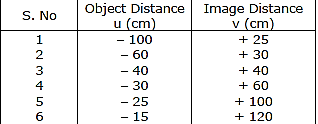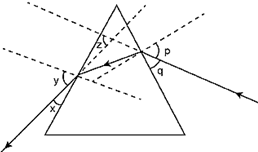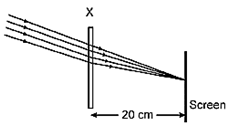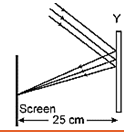### CBSE Class 10 Science Previous Year Paper 2017# CBSE CLASS 10 SCIENCE PREVIOUS YEAR PAPER 2017

CBSE Board

Class 10th Science

Previous year paper

2017

# Time: 3 hrs.                                                                                                                      Max. Marks: 90

Instructions:

1. The question paper comprises two Sections, A and B. You are to attempt both the sections.

2. All questions are compulsory.

3. There is no choice in any of the questions.

4. All questions of Section A and all questions of Section B are to be attempted separately.

5. Question numbers 1 to 3 in Section A are one-mark questions. These are to be answered in one word or in one sentence.

6. Question numbers 4 to 6 in Section A are two-mark questions. These are to be answered in about 30 words each.

7. Question numbers 7 to 18 in Section A are three-mark questions. These are to be answered in about 50 words each.

8. Question numbers 19 to 24 in Section A are five-mark questions. These are to be answered in about 70 words each.

9. Question numbers 25 to 33 in Section B are multiple choice questions based on practical skills. Each question is a one-mark question. You are to select one most appropriate response out of the four provided to you.

10. Question numbers 34 to 36 in Section B are two-marks questions based on practical skills. These are to be answered in brief.

SECTION A

Question 1. Write the molecular formula of the 2nd and 3rd member of the homologous series where the first member is ethyne.

Question 2. Why is variation important for a species?

Question 3. In the following food chain, 20,000 J of energy was available to the plants. How much energy would be available to man in this chain?

Plants →Sheep → Man

Question 4. An object is placed at a distance of 15 cm from a concave lens of focal length 30 cm. List four characteristic (nature, position, etc.) of the image formed by the lens.

Question 5. You being an environmentalist are interested in contributing towards the conservation of nature resources. List four activities that you can do on your own.

Question 6. Why are coal and petroleum categorized as natural resources? Given a reason as to why they should be used judiciously.

Question 7. Distinguish between esterification and saponification reactions with the help of the chemical equations for each. State one use of each (i) esters, and (ii) saponification process.

Question 8. Write the structural formula of ethanol. What happens when it is heated with excess of conc. H2SO4 at 443 K? Write the chemical equation for the reaction stating the role of conc. H2SO4 in this reaction.

Question 9. What is periodicity in properties of elements with reference to the Modern Periodic Table? Why do all the elements of the same group have similar properties? How does the tendency of elements to gain electrons change as we move from left to right in a period? State the reason of this change?

Question 10. Write the electronic configuration two elements X and Y whose atomic numbers are 20 and 17 respectively. Write the Molecular formula of the compound formed when element. X reacts with element Y. Draw electron-dot structure of the product and also state the nature of the bond formed between both the elements.

Question 11. How did Mendel explain that it is possible that a trait is inherited but not expressed in an organism?

Question 12. What is an organic evolution? It cannot be equated with progress. Explain with the help of a suitable example.

Question 13. List the two types of reproduction. Which one of the two is responsible for bringing in more variations in its progeny and how?

Question 14. What is vegetative propagation? State two advantages and two disadvantages of this method.

Question 15. List three techniques that have been developed to prevent pregnancy. Which one of these techniques is not meant for males? How does the use of these techniques have a direct impact on the health and prosperity of a family?

Question 16. “A lens can form a magnified erect image as well as magnified inverted image of an object placed in front of it”. State the nature of this lens and draw ray diagrams to justify the above statement. Mark the positions of O, F and 2F in the diagram.

Question 17. What is “dispersion of white light”? Draw a labelled diagram to illustrate the recombination of the spectrum of white light. Why it is essential that the two prisms used for the purpose should be identical and placed in an inverted position with respect to each other?

Question 18. (a) Water is an elixir of life a very important natural resource. Your science teacher wants you to prepare a plan for a formative assessment activity, “How to save water, the vital natural resource”. Write any two ways that you will suggest to bring awareness in your neighborhood, on how to save water’.

(b) Name and explain any one way by which the underground water table does not go down further.

Question 19. With the help of one example for each, distinguish between the acquired traits and the inherited traits. Why are the traits/experiences acquired during the entire lifetime of an individual not inherited in the next generation? Explain the reason of this fact with an example.

Question 20. (a) Write the functions of each of the following parts in a human female reproductive system:

1. Ovary

2. Uterus

3. Fallopian tube

(b) Write the structure and functions of placenta in a human female.

Question 21. Why certain compounds are called hydrocarbons? Write the general formula for homologous series of alkanes, alkenes and alkynes and also draw the structure of the first member of each series. Write the name of the reaction that converts alkenes into alkanes and also write a chemical equation to show the necessary conditions for the reaction to occur.

Question 22. (a) A student suffering from myopia is not able to see distinctly the object placed beyond 5 m. List two possible reasons due to which this defect of vision may have arisen. With the help of ray diagrams explain.

1. Why the student is unable to see distinctly the objects placed beyond 5 cm from his eyes.

2. The type of the corrective lens used to restore proper vision and how this defect is corrected by the use of this lens.

(b) If, in this case, the numerical value of the focal length of the corrective lens is 5 m, find the power of the lens as per the new Cartesian sign convention.

Question 23. Analyse the following observation table showing variation of image distance (v) with object distance (u) in case of a convex lens and answer the questions that follow without doing any calculations:(a) What is the focal length of the convex lens? Give reason to justify your answer.

(b) Write the serial number of the observation which is not correct. On what basis have you arrived at this conclusion?

(c) Select an appropriate scale and draw a ray diagram for the observation at S. No. 2. Also find the approximate value of magnification.

Question 24. (a) If the image formed by a mirror for all position of the object placed in front of it is always diminished, erect and virtual, state the type of the mirror and also draw a ray diagram to justify your answer. Write one use such mirrors are put to and why.

(b) Define the radius of curvature of spherical mirrors. Find the nature and focal length of a spherical mirror whose radius of curvature is +24 cm

SECTION B

Question 25. Study the following ray diagram:In this diagram, the angle of incidence, the angle of emergence and the angle of deviation respectively have been represented by

1. y, p, z

2. x, q, z

3. p, y, z

4. P, z, y

Question 26. A student very cautiously traces the path of a ray through a glass slab for different values of the angle of incidence ( $angle$ i). He then measures the corresponding values of the angle of refraction ( $angle$ r) and the angle of emergence ( $angle$ e) for every value of the angle of incidence. On analysing these measurements of angles, his conclusion would be

1. $angle$ i > $angle$ r > $angle$e

2. $angle$ i = $angle$ e > $angle$ r

3. $angle$ i < $angle$ r < $angle$ e

4. $angle$ i = $angle$ e < $angle$ r

Question 27. Study the given ray diagrams and select the correct statement from the following:1. (A) Device X is a concave mirror and device Y is a convex lens, whose focal lengths are 20 cm and 25 cm respectively.

2. Device X is a convex lens and device Y is a concave mirror, whose focal lengths are 10 cm and 25 cm respectively

3. Device X is a concave lens and device Y is a convex mirror, whose focal lengths are 20 cm and 25 cm respectively.

4. Device X is a convex lens and device Y is a concave mirror, whose focal lengths are 20 cm and 25 cm respectively.

Question 28. A student obtains a blurred image of a distant object on a screen using a convex lens. To obtain a distinct image on the screen he should move the lens.

1. away from the screen

2. towards the screen

3. to a position very far away from the screen

4. either towards or away from the screen depending upon the position of the object.

Question 29. While studying the saponification reaction, what do you observe when you mix an equal amount of colorless vegetable oil and 20% aqueous solution of NaOH in a beaker?

1. The color of the mixture has become dark brown

2. A brisk effervescence is taking place in the beaker

3. The outer surface of the beaker has become hot

4. The outer surface of the beaker has become cold

Question 30. When you add a few drops of acetic acid to a test-tube containing sodium bicarbonate powder, which one of the following is your observation?

1. No reaction takes place

2. A colorless gas with pungent smell is released with brisk effervescence

3. A brown colored gas is released with brisk effervescence

4. Formation of bubbles of a colorless and odorless gas

Question 31. A student requires hard water for an experiment in his laboratory which is not available in the  neighboring area. In the laboratory there are some salts, which when dissolved in distilled water can convert it into hard water. Select from the following groups of salts, a group, each salt of which when dissolved in distilled water will make it hard.

1. Sodium chloride, Potassium chloride

2. Sodium sulphate, Potassium sulphate

3. Sodium sulphate, Calcium sulphate

4. Calcium sulphate, Calcium chloride

Question 32. To perform an experiment to identify the different parts of an embryo of a dicot seed, first of all you require a dicot seed. Select dicot seeds from the following group:

Wheat, Gram, Maize, Pea, Barley, Ground-nut

1. Wheat, Gram and Pea

2. Gram, Pea and Ground-nut

3. Maize, Pea and Barley

4. Gram, Maize and Ground-nut

Question 33. The following vegetables are kept in a basket

Potato, Tomato, Radish, Brinjal, Carrot, Bottle-gourd

Which two of these vegetables correctly represent the homologous structures?

1. Carrot and Tomato

2. Potato and Brinjal

Question 34. Draw in sequence (showing the four stages), the process of binary fission in Amoeba.

Question 35. A student focuses the image of a candle flame, placed at about 2 m from a convex lens of focal length 10 cm, on a screen. After that he moves gradually the flame towards the lens and each time focuses its image on the screen.

1. In which direction does he move the lens to focus the flame on the screen?

2. What happens to the size of the image of the flame formed on the screen?

3. What difference is seen in the intensity (brightness) of the image of the flame on the screen?

4. What is seen on the screen when the flame is very close (at about 5 cm) to the lens?

Question 36. Mention the essential material (chemicals) to prepare soap in the laboratory. Describe in brief the test of determining the nature (acidic/alkaline) of the reaction mixture of saponification reaction.

Privacy Settings